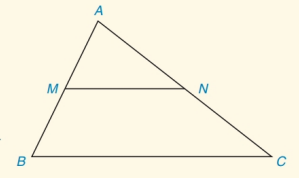Chapter 4.CT, Problem 8CT### Elementary Geometry for College St...

6th Edition
Daniel C. Alexander + 1 other
ISBN: 9781285195698

#### Solutions

Chapter
Section### Elementary Geometry for College St...

6th Edition
Daniel C. Alexander + 1 other
ISBN: 9781285195698
Textbook Problem
1 views

# In Δ A B C , M is the midpoint of A B ¯ and N is the midpoint if A C ¯ .a) How are the line segments M N ¯   and  B C ¯ related?                                     _ b) Use an equation to state how the lengths MN and BC are related.                                     _To determine

(a)

To Find:

MN¯and BC¯ are related.

Explanation

Given:

M is the midpoint of AB¯ and N is the midpoint of AC¯.

Approach:

Since, M is the midpoint of AB¯ and N is the midpoint of AC¯

To determine

(b)

To Find:

The equation to show the relation between MN and BC.

### Still sussing out bartleby?

Check out a sample textbook solution.

See a sample solution

#### The Solution to Your Study Problems

Bartleby provides explanations to thousands of textbook problems written by our experts, many with advanced degrees!

Get Started

#### Convert the expressions in Exercises 6584 to power form. 3x453x+43xx

Finite Mathematics and Applied Calculus (MindTap Course List)

#### The graph of is: a) b) c) d)

Study Guide for Stewart's Multivariable Calculus, 8th

#### True or False: f(x) is symmetric about the origin if f(x) =f(x).

Study Guide for Stewart's Single Variable Calculus: Early Transcendentals, 8th